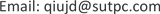1. 引言

2. 数据来源

2) 训练指纹坐标：一个697 × 3的矩阵(x,y,z的3D坐标)；

4) 测试指纹坐标：一个3951 × 3的矩阵(x,y,z的3D坐标)。

3. 研究方法3.1. 三角算法

1) 建立信标节点与未知节点距离方程组

{ ( x 1 − x ) 2 + ( y 1 − y ) 2 = d 1 2 ⋮ ( x n − x ) 2 + ( y n − y ) 2 = d n 2

2) 上边方程组为非线性方程组，用方程组中前n − 1个方程减去第n个方程后，得到线性化的方程：

A * X = b

A = [ 2 ( x 1 − x n ) ⋯ 2 ( y 1 − y n ) ⋮ ⋱ ⋮ 2 ( x n − 1 − x n ) ⋯ 2 ( y n − 1 − y n ) ] , b = [ x 1 2 − x n 2 + y 1 2 − y n 2 + d n 2 − d 1 2 ⋮ x n − 1 2 − x n 2 + y n − 1 2 − y n 2 + d n 2 − d n − 1 2 ]

3) 用最小二乘法求解上边方程得：

X = ( A T A ) − 1 A T b

X便是未知终端的坐标计算值。

3.2. 位置指纹算法

D = ∑ i = 1 N | r i − ρ i | 2

4. 基于Wi-Fi指纹的室内定位算法实现

1) 先进行楼层分类再进行每个楼层的具体定位，将三维的误差降低到二维误差，部分提高定位精度，大大降低了计算量。

2) 使用神经网络算法训练模型进行楼层定位，模型构成后只需要花费少量时间就可以得到分类的结果，减少了分类计算的时间，具有良好的时效性。

4.1. 楼层分类4.1.1. 自动编码器模型

4.1.2. 神经网络模型

4.1.3. 楼层分类模型

4.2. 单楼层定位

3) 根据权值估算测试指纹坐标 Y = ∑ i = 1 K X i * W i ∑ i = 1 K W i ，X为训练指纹坐标，Y为估算坐标。通过使用加权K近

5. 实验结果

Comparisons of algorithm

KNN87%8.09

6. 结论

1) 基于神经网络的楼层分类模型加上加权KNN算法在时效性上要优于各个传统Wi-Fi指纹室内定位算法。

2) 实验结果表明基于神经网络的楼层分类模型可以实现坐标与对应楼层的快速匹配，使用加权KNN算法对单个楼层坐标可以进行更高精度的具体定位，楼层分类准确率在四个算法中最高，达到92%，同时多次定位的实验精度在4米左右，效果较好，往后可以应用于大型室内导航。